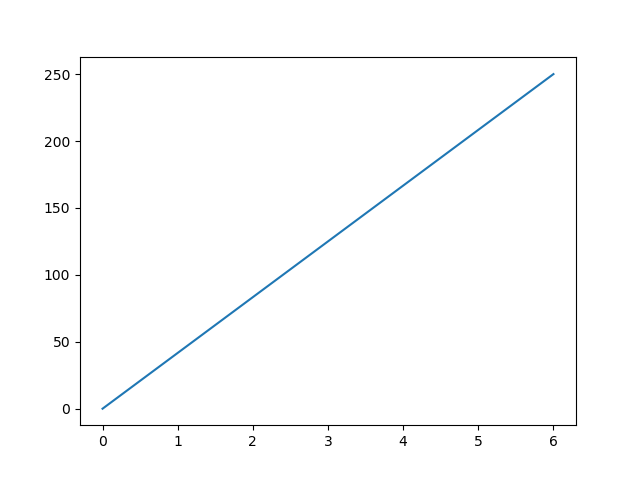THE WORLD'S LARGEST WEB DEVELOPER SITE

# Matplotlib Pyplot

## Pyplot

Most of the Matplotlib utilities lies under the `pyplot` submodule, and are usually imported under the `plt` alias:

import matplotlib.pyplot as plt

Now the Pyplot package can be referred to as `plt`.

### Example

Draw a line in a diagram from position (0,0) to position (6,250):

import matplotlib.pyplot as plt
import numpy as np

xpoints = np.array([0, 6])
ypoints = np.array([0, 250])

plt.plot(xpoints, ypoints)
plt.show()

### Result:Try it Yourself »